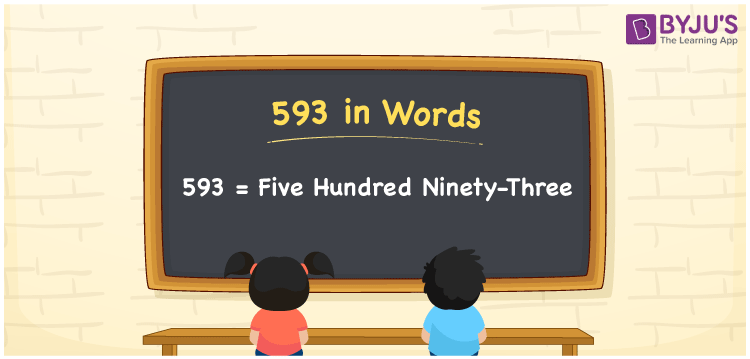# 593 in Words

593 in words is written as Five hundred ninety-three In both the International System of Numerals and the Indian System of Numerals, 593 is written as Five hundred ninety-three The number 593 is a Cardinal Number as it represents some quantity. For example, the “cost of the dress is 593 rupees”.

 593 in Words Nine hundred seventy-three Nine hundred seventy-three in Number 593

## 593 in English Words

We write 593 in English Words using the letters of the English alphabet. Therefore, we read 593 in English as “Nine hundred seventy-three”.## How to Write 593 in Words?

To write 593 in words, we shall use the place value chart. In the place value chart, write 5 in the hundreds, 9 in the tens, and 3 in the ones, respectively. Now let us make a place value chart to write the number 593 in words.

 Hundreds Tens Ones 5 9 3

Thus, we can write the expanded form as

5 × Hundred + 9 × Ten + 3 × One

= 5 × 100 + 9 × 10 + 3 × 1

= 500 + 90 + 3

= 593

= Five hundred ninety-three

593 is a natural number, the successor of 592 and the predecessor of 594.

593 in words – Nine hundred seventy-three

• Is 593 an odd number? – Yes
• Is 593 an even number? – No
• Is 593 a perfect square number? – No
• Is 593 a perfect cube number? – No
• Is 593 a prime number? – Yes
• Is 593 a composite number? – No

## Frequently Asked Questions on 593 in Words

Q1

### How to write 593 in words?

593 in words is written as Five hundred ninety-three
Q2

### How to write 593 in the International and Indian System of Numerals?

In both, the system of numerals, 593 in words, is written as Five hundred ninety-three
Q3

### What is the successor of 593?

The successor of 593 is 594.